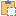#### Grid Class

Represents a single grid line within Autodesk Revit.

Namespace: Autodesk.Revit.DB
Assembly: RevitAPI (in RevitAPI.dll) Version: 18.0.0.0 (18.0.0.420)

# Syntax

C#
``public class Grid : DatumPlane``
Visual Basic
``````Public Class Grid _
Inherits DatumPlane``````
Visual C++
``public ref class Grid : public DatumPlane``

# Remarks

A Grid is a DatumPlane, so it is actually a three dimensional surface. It can be either a plane parallel to the project z-axis, or else a cylinder whose axis is parallel to the project z-xis. [!:Autodesk::Revit::DatumPlane]

# ExamplesCopy C#
``````public void GetInfo_Grid(Grid grid)
{
string message = "Grid : ";

// Show IsCurved property
message += "\nIf grid is Arc : " + grid.IsCurved;

// Show Curve information
Autodesk.Revit.DB.Curve curve = grid.Curve;
if (grid.IsCurved)
{
// if the curve is an arc, give center and radius information
Autodesk.Revit.DB.Arc arc = curve as Autodesk.Revit.DB.Arc;
message += "\nArc's center:  (" + XYZToString(arc.Center);
}
else
{
// if the curve is a line, give length information
Autodesk.Revit.DB.Line line = curve as Autodesk.Revit.DB.Line;
message += "\nLine's Length: " + line.Length;
}
// Get curve start point
message += "\nStart point: " + XYZToString(curve.GetEndPoint(0));
// Get curve end point
message += "; End point: " + XYZToString(curve.GetEndPoint(0));

}

// output the point's three coordinates
string XYZToString(XYZ point)
{
return "(" + point.X + ", " + point.Y + ", " + point.Z + ")";
}``````Copy VB.NET
``````Public Sub GetInfo_Grid(grid As Grid)
Dim message As String = "Grid : "

' Show IsCurved property
message += vbLf & "If grid is Arc : " & Convert.ToString(grid.IsCurved)

' Show Curve information
Dim curve As Autodesk.Revit.DB.Curve = grid.Curve
If grid.IsCurved Then
' if the curve is an arc, give center and radius information
Dim arc As Autodesk.Revit.DB.Arc = TryCast(curve, Autodesk.Revit.DB.Arc)
message += vbLf & "Arc's center:  (" & XYZToString(arc.Center)
Else
' if the curve is a line, give length information
Dim line As Autodesk.Revit.DB.Line = TryCast(curve, Autodesk.Revit.DB.Line)
message += vbLf & "Line's Length: " + line.Length
End If
' Get curve start point
message += vbLf & "Start point: " & XYZToString(curve.GetEndPoint(0))
' Get curve end point
message += "; End point: " & XYZToString(curve.GetEndPoint(0))